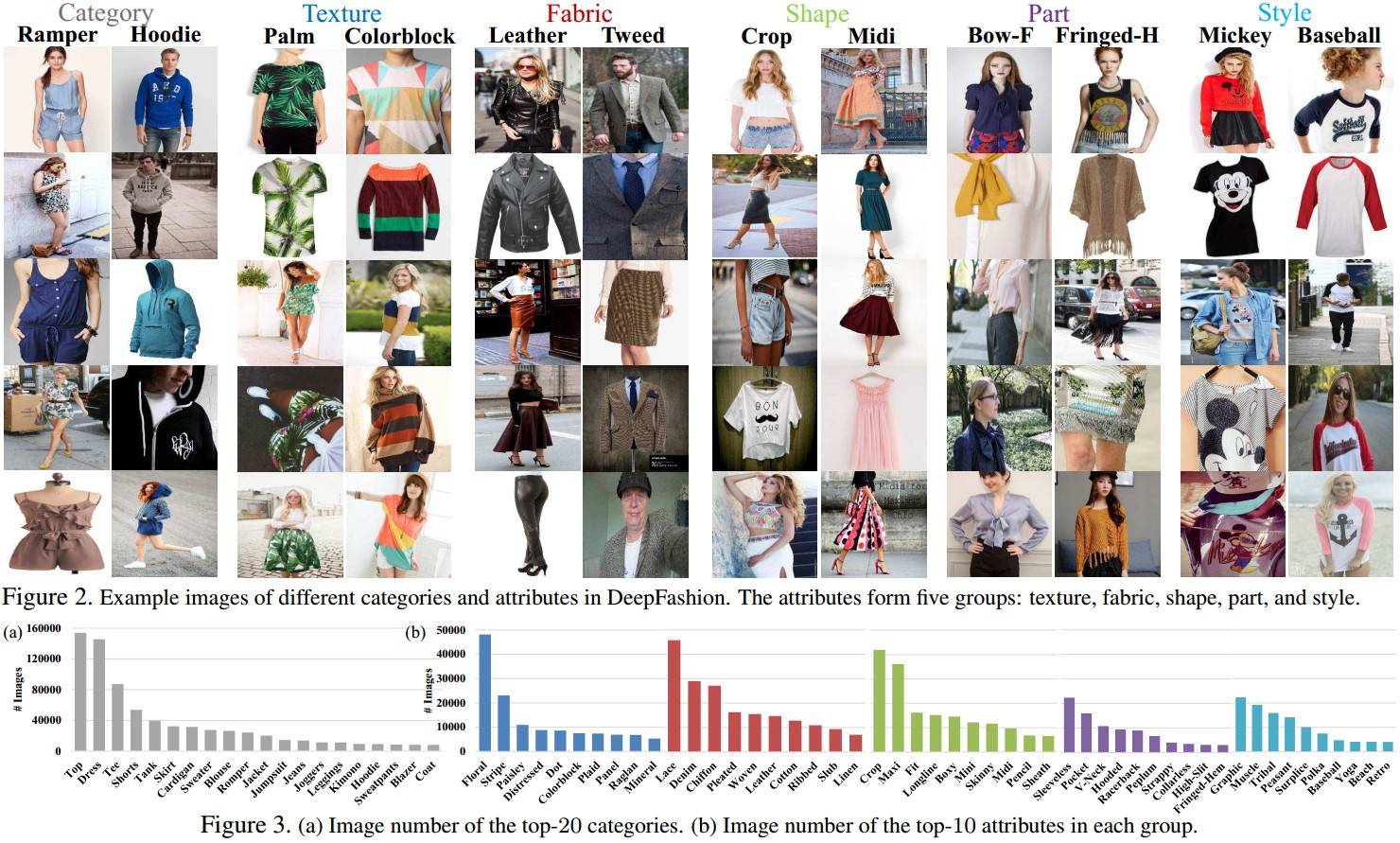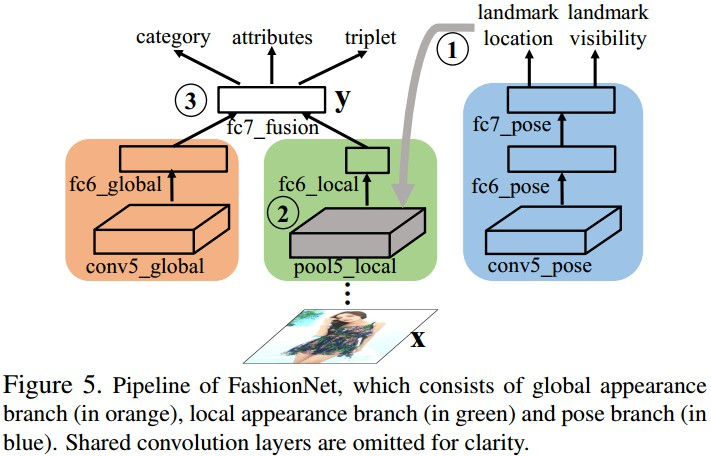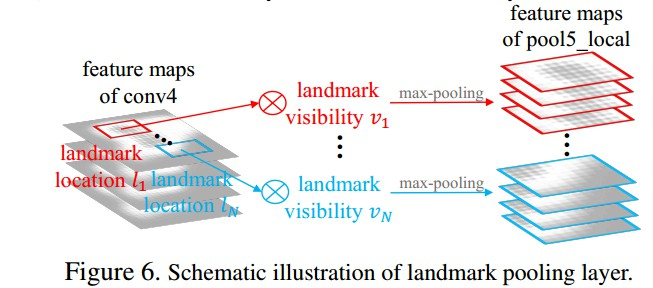[DeepFashion - Project]

[DeepFashion - Dataset]

[fashion-detection-Code]

<h2>1. DeepFashion Dataset</h2>

• 综合性强(Comprehensiveness) —— 标注信息包括：50 fine-grained categories, 1000 attributes, landmarks, bounding box, 300000 cross-pose/cross-domain pair correspondences.
• 规模大(Scale) —— 超过 800K 标注的服装图像
• 可利用性好(Availability) —— 对研究性应用公开.• 大规模属性(Massive attributes) - 对于服装商品识别必须的信息；
• 关键点(Landmarks) - 关键点位置能够有效处理形变和姿态变化；
• 买家与商家对(Consumer-to-shop pairs) - 建立 cross-domain gap 的桥梁.

DeepFashon 数据集用途：

• 类别与属性预测(Category and Attribute Prediction) 该任务是对 50 个fine-gained 类别和 1000 个属性进行分类. 共 63720 张标注图片.
• 对于类别分类，采用标准的 top-k 分类精度 作为评价准则； 1-of-K classification problem.
• 对于属性预测，采用 top-k recall rate 作为评价准则，通过对 1000 个分类 scores 进行排名，检测在 top-k 中所匹配的属性数量； multi-label tagging problem.
• 商家服装检索(In-Shop Clothes Retrieval) 该任务是判断两张商家图像是否属于同一款. 共 11735 款服装，54642 张图片(From Forever21). 采用 top-k 检索精度作为评价准则，如果在 top-k 检索结果中能够精确找到服装款，则认为是检索正确.</p>
• 买家到商家服装检索(Consumer-to-Shop Clothes Retrieval) 该任务是匹配买家所拍照片与商家的服装. 251361 张买家与商家对{From Mogujie). 采用 top-k 检索精度作为评价准则.</p>

<h2>2. FashionNet</h2>

<h3>2.1 网络结构</h3>Figure 5. FashionNet 结构. 包括全局特征分支(橙色部分)，局部特征分支(绿色部分)，pose 分支(蓝色分支). 其中，忽略了共享卷积层.

Pose分支—— 预测关键点位置及其可见性(可见性指，关键点是否存在).

<h3>2.2 前向传播(Forward Pass)</h3>

FusionNet 主要包括三个阶段：

• Stage 1 - 服装图像输入网络，并在蓝色分支传递，以预测关键点位置；
• Stage 2 - 在 pool5_local 层对估计的关键点进行池化(pooling or gate)特征，以使布局特征对服装形变和缺失具有不变性；
• Stage 3 - 在 fc7_fusion 对 fc6_global 层全局特征和 fc6_local池化后的关键点局部特征进行连接.

<h3>2.3 反向传播(Backward Pass)</h3>

FusionNet 有四种损失函数，以迭代方式进行.

• 关键点定位的 regression loss； L2 regression loss： ${ L_{landmarks} = \sum _{j=1} ^{|D|} ||\mathbf{v}_j \cdot (\check{l}_j- l_j)||_2^2 }$ ${ D }$ - 训练样本数； ${ \check{l}_j }$ - 第 j 个样本的 groundtruth 关键点位置； ${ \mathbf{v}_j }$ - 第 j 个样本的关键点可见性向量，1 表示可见，0 表示不可见； 这里，关键点可见性变量可以纠正关键点 groundtruth 位置的丢失，如果某个关键点丢失的化，该erro则不进行反向传播.
• 关键点可见性和服装类别预测的 softmax loss； 1-of-K softmax loss 来对关键点可见性和 fine-grained 类别进行分类， 分别记为 ${L_{visibility} }$ 和 ${L_{category} }$.
• 属性预测的 cross-entropy loss； 加权 cross-entropy loss： ${ L_{attributes} = \sum_{j=1}^{|D|} (w_{pos} \cdot \mathbf{a}_j log p(\mathbf{a}_j | \mathbf{x}_j) + w _{neg} \cdot (1- \mathbf{a}_j)log(1- p(\mathbf{a}_j | \mathbf{x}_j))) }$ 其中， ${ \mathbf{x}_j }$ 和 ${ \mathbf{a}_j }$ 分别表示第 j 张服装图像及对应的属性标签； ${ w_{pos} }$ 和 ${ w_{neg} }$ 为两个系数，由训练集中 positive 和 negetive 样本数量的比率确定. ​
• 成对(pairwise)服装图像度量学习的 triplet loss 服装图像对的度量采用 triplet loss，增强 positive 和 negetive 样本的距离约束： ${ L_{triplet} = \sum _{j=1}^{|D|} max&#123;0, m+d(\mathbf{x}_j, \mathbf{x}_j^{+})- d(\mathbf{x}_j, \mathbf{x}_j^{-})&#125; }$ 其中， ${ (\mathbf{x}_j, \mathbf{x}_j^{+}, \mathbf{x}_j^{-}) }$ 为一个三元组，${ \mathbf{x}^{+} }$ 和 ${ \mathbf{x}^{-} }$ 分别表示关于图像 ${ \mathbf{x} }$ 的相同款图像与不同款图像. ${ d(\cdot, \cdot) }$ 为距离函数； ${ m }$ 为 边缘参数(margin parameter). ​

• Step 1 - 蓝色分支作为主要task，其它分支作为辅助 tasks. 对 ${ L_{visibility} }$ 和 ${ L_{landmark} }$ 赋予较大权重，其它 loss 赋予较小权重. 由于其它任务和关键点估计的相关性，故可以利用其它任务来辅助训练关键点估计任务.
• Step 2 - 预测服装类别和属性，同时学习服装图像的成对关联性(pairwise relations). 这里利用估计的关键点位置来池化(pool) 局部特征.

<h3>2.4 关键点池化层(Landmark Pooling Layer)</h3>landmark pooling 层的输入是 feature maps(如 conv4 ) 和 估计的 landmarks.

landmark pooling 层的反向传播类似于 Fast R-CNN中的 RoI pooling 层. 不同之处在于，Fast R-CNN中的 RoI 层将池化的区域(pooled regions) 独立对待，landmark pooling 层通过连接局部特征来捕捉不同服装关键点的交互关联性(interaction).

Last modification：October 10th, 2018 at 04:27 pm